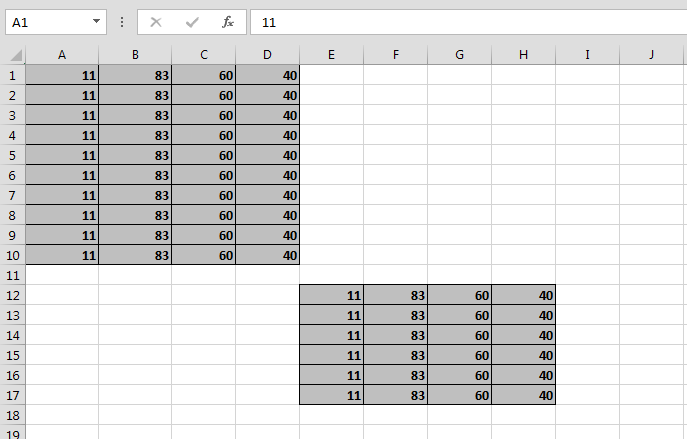# Change range with more then Area to values using VBA in Microsoft Excel

In this article you will learn about how to change range with more than area to values using VBA in Microsoft Excel.

Let’s take an example and understand how to write the VBA code to change the range with more than area to values.

How to change range with more than area to values?

Example 1:-

We have data in Excel A1:C1, in this data we want to paste in the different ranges. Let’s see how?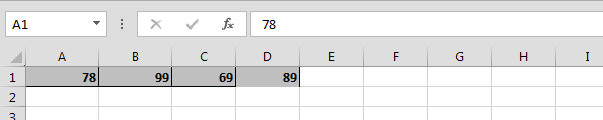• Press Alt+F11 key to open the Visual Basic Application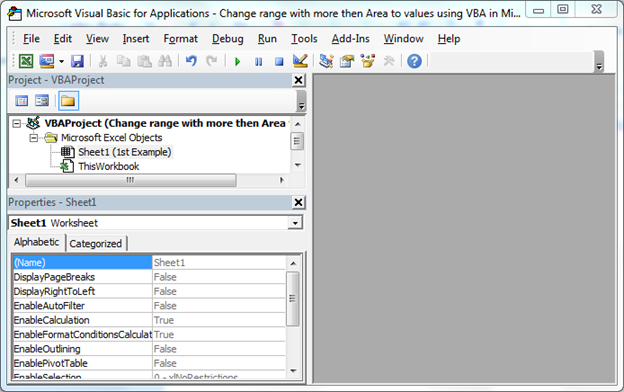• In VBAProject Double click on Sheet 1
• Enter the below given VBA Code

```Sub Values_4()

Dim smallrng As Range

For Each smallrng In Range("A1:D10,E12:H17").Areas

smallrng.Value = Range("A1:D1").Value

Next

End Sub

```
• To run the code press F5 key
• Values will be updated in defined small range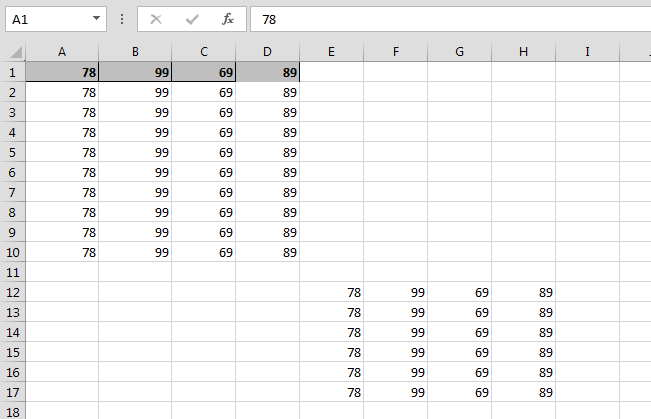Example 2:-

Let’s say we have data in the defined small range and we want to over write that data with the particular range data.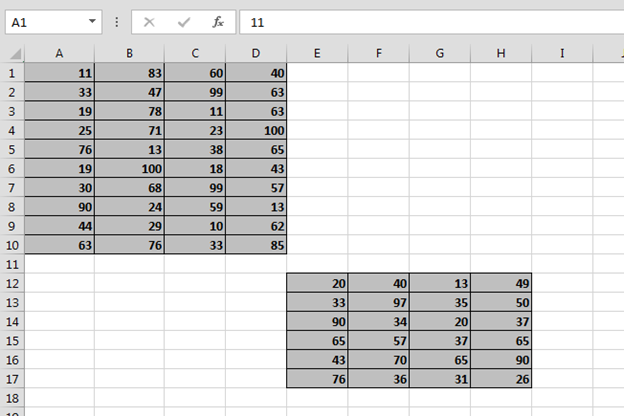To solve this problem follow below given steps:-

• Press the key Alt+F11 and double click on sheet 2
• Enter the below code:-

```Sub Values_4()

Dim smallrng As Range

For Each smallrng In Range("A1:D10,E12:H17").Areas

smallrng.Value = Range("A1:D1").Value

Next

End Sub
```

• Run the code by pressing F5
• Values will get updated